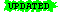Knowledge Representation & Reasoning
Discrete Structures

# Representing English Sentences in FOL

 Last Update: 27 February 2005 Note:ormaterial is highlighted

This document uses "pretend-it's-English" semantics, for convenience only :-)

EnglishFOL
Mark Twain is wiseWise(mt)
Mark Twain is Samuel Clemensmt = sc
All dogs are animalsx[Dog(x)Animal(x)]
Some dogs are pets.x[Dog(x) ^ Pet(x)]
No dogs are fish.¬x[Dog(x) ^ Fish(x)]
All dogs are not fish.x[Dog(x)¬Fish(x)]
Some dogs are not pets.x[Dog(x) ^ ¬Pet(x)]
No dogs are not mammals.¬x[Dog(x) ^ ¬Mammal(x)]
Not all dogs are pets.¬x[Dog(x)Pet(x)]
All that glitters is not gold.¬x[Glitters(x)Gold(x)] (!)
Every boy loves a girl.x[Boy(x)y[Girl(y) ^ Loves(x,y)]]
(There is) A girl (who) is loved by every boy.y[Girl(y) ^x[Boy(x)Loves(x,y)]]
Everyone loves someone.xy.Loves(x,y)
Someone is loved by everyone.yx.Loves(x,y)
There is exactly one P.x[P(x) ^y[P(y)y=x]]
The present King of France is bald.x[is_a_present_king_of_France(x) ^y[is_a_present_king_of_France(y)y=x] ^ Bald(x)]
The present King of France is not bald.x[is_a_present_king_of_France(x) ^y[is_a_present_king_of_France(y)y=x] ^ ¬Bald(x)]
The present King of France is not bald.¬x[is_a_present_king_of_France(x) ^y[is_a_present_king_of_France(y)y=x] ^ Bald(x)]
John goes to school.Goes(john, school) (?)
John goes to school.Goes(john-3, school-173) (?)
John goes to school.x[Named(x, "John") ^y[School(y) ^ Goes(x,y)]]
orxy[Named(x, "John") ^ School(y) ^ Goes(x,y)]

Need an ontology of names!

John goes to school.above, conjoined with:
Person(x) ^ Male(x) ^z[School(z)...]
John sold a book to Mary.xyz[Named(x, "John") ^ Named(y, "Mary") ^ Book(z) ^ Sold(x,y,z)]
John sold a book to Mary.xyzt[Time(t) ^ Named(x, "John") ^ Named(y, "Mary") ^ Book(z) ^ Sell(x,y,z,t) ^ Before(t,n) ^ Now(n)] (?)

Need an ontology of time!

Mary bought a book from John.As above
and/orxyz[Sold(x,y,z)Buy(y,x,z)]